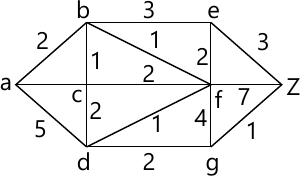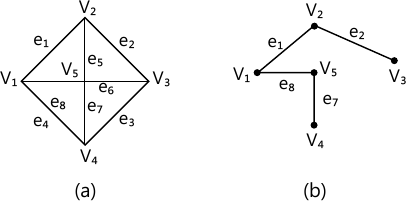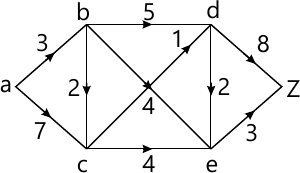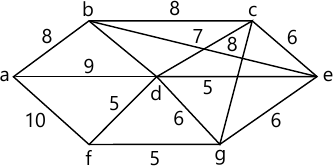MORE IN Discrete Mathematics
SPPU Computer Engineering (Semester 3)
Discrete Mathematics
December 2014
Total marks: --
Total time: --
INSTRUCTIONS
(1) Assume appropriate data and state your reasons
(2) Marks are given to the right of every question
(3) Draw neat diagrams wherever necessary

Answer any one question from Q1 and Q2
1 (a) Find DNF of ((p→q) ∩ (q→p)) ∨ p.
Find CNF of p ↔ (˜ p ∨ ˜ q)
4 M
1 (b) Consider a set of integers 1 to 500. Find how many if these numbers are divisible by 3 or by 5 or by 11.
2 M
1 (c) Show that the set of all divisors of 36 forms a lattice.
6 M

2 (a) Explain concept of countably infinite set with example.
3 M
2 (b) Use mathematical induction to show that : n(n2 ? 1) is divisible by 24 where n is any odd +ve number.
3 M
2 (c) Consider the following relation on {1, 2, 3, 4, 5, 6} : R = {(i,j) : |i - j |= 2}. Is R transitive? Is R reflexive? Is R symmetric ?
2 M
2 (d) Let R be the relation on the set A = {a, b, c, d, e, f} and R = {(a, c), (b, d), (c, a), (c, e), (d, b), (d, f), (e, c), (f, d)}. Find the transitive closure of R using Warshall's algorithm.
4 M

Answer any one question from Q3 and Q4
3 (a) (i) Prove that every cyclic group is an abelian group.
3 M
3 (a) (ii) Explain Group Homomorphism and Isomorphism of Group. Take suitable example.
3 M
3 (b) (i) Under what condition Km, n will have Eulerian circuit ?
4 M
3 (b) (ii) How many colours are required to colour Km, n. ? Why ?
2 M

4 (a) Prove that : $(a+b \sqrt{2}, _, *)$ where a, b ∈ R is integral domain.
6 M
4 (b) Find the shortest path between a-z for the graph given in figure below using Dijkstra's algorithm.6 M

Answer any one question from Q5 and Q6
5 (a) Prove that the number of vertices is one more than the number of edges in a tree.
3 M
5 (b) Find out fundamental cut-sets of the following graph with respect to given spanning tree:3 M
5 (c) Use labelling procedure to find a maximum flow in the transport network given in the following figure. Determine the corresponding minimum cut.7 M

6 (a) Find minimum spanning tree for graph given in the following figure using Prim's algorithm.7 M
6 (b) For the following set of weights, construct optimal binary prefix code:
 α 5 β 6 γ 6 δ 11 ε 20
4 M
6 (c) Define rooted tree.
2 M

Answer any one question from Q7 and Q8
7 (a) A box contains 6 white and 6 black balls. Find number of ways 4 balls can be drawn from the box if
(i) Two must be white
(ii) All of them must have same colour.
6 M
7 (b) Two computers A and B are to be marketed. A salesman who is assigned the job of finding customers for them has 60 percent and 40 percent chances respectively of succeeding in case of computer A and B. The two computers can be sold independently. Given that he was able to sell at least one computer, what is the probability that computer A has been sold ?
7 M

8 (a) Out of 5 males and 6 females a committee of 5 is to be formed. Find the number of ways in which it can be formed so that among the person chosen in the committee there are:
(i) exactly 3 males and 2 females
(ii) at least 2 males and one female.
6 M
8 (b) The probability that a trainee will remain in a company is 0.6. The probability that the employee earns more than Rs. 10,000 per month is 0.5. The probability that an employee is a trainee who remains within the company or who earns more than Rs. 10,000 per month is 0.7. What is the probability that an employee earns more than Rs. 10,000 per month ? Given that he is a trainee who stayed with the company.
7 M

More question papers from Discrete Mathematics